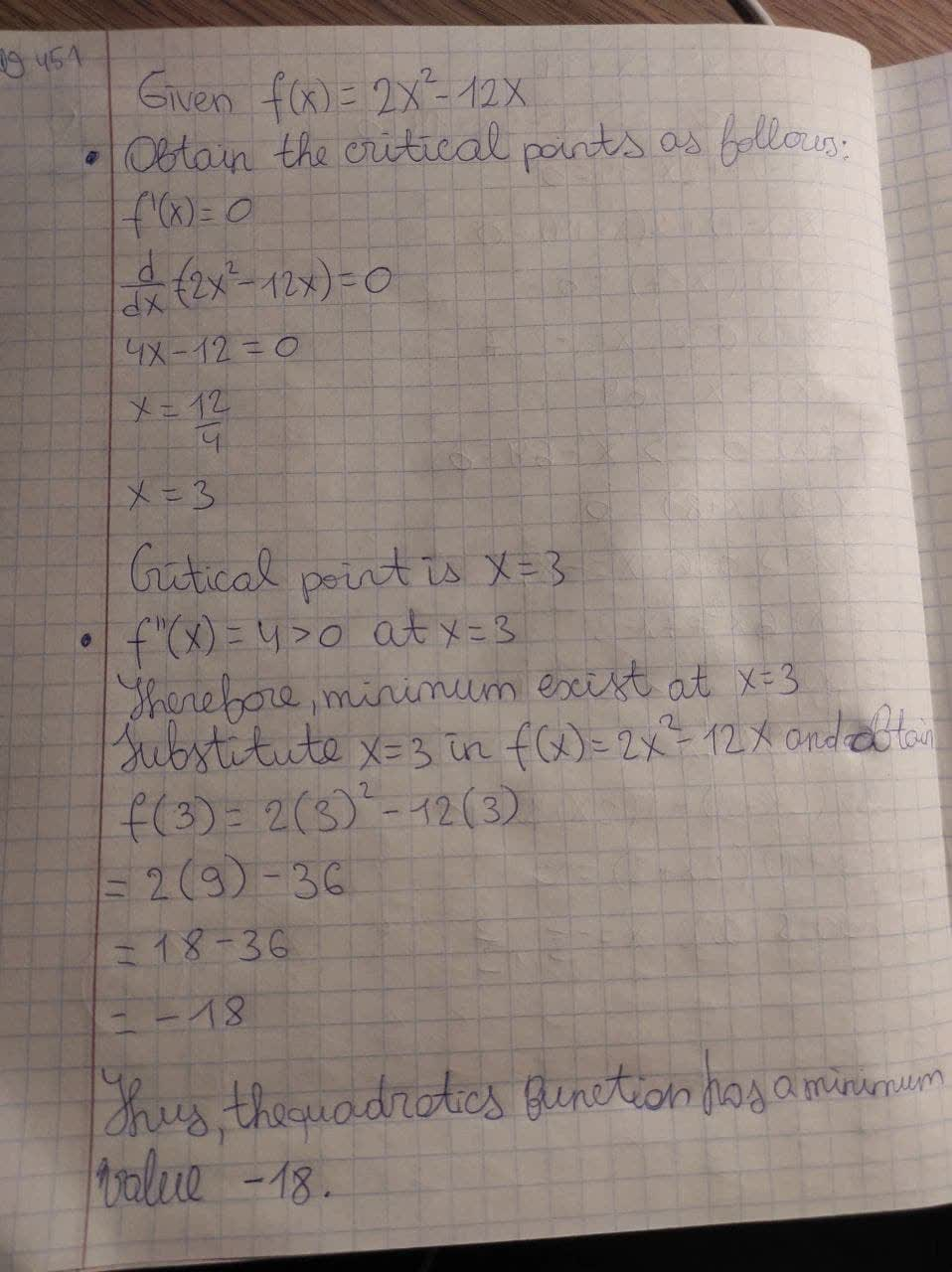# Deetermine, without graphing, ehether the given quaratic function has a maximum value or a minimum value and then find the valur.f(x) = 2x^2-12xsagnuhh 2021-03-09 Answered

Deetermine, without graphing, ehether the given quaratic function has a maximum value or a minimum value and then find the valur. $$f(x) = 2x^2-12x$$

• Questions are typically answered in as fast as 30 minutes

### Plainmath recommends

• Get a detailed answer even on the hardest topics.
• Ask an expert for a step-by-step guidance to learn to do it yourself.Leonard Stokes

Obtain the critical points as follows. $$f'(x)=0 \frac{d}{dx}(2x^2-12x)=0\ 4x-12=0 x=\frac{12}{4} x=3$$ Thus, the critical point is $$x=3$$ $$f''(x)=4>0$$ at $$x=3$$ Therefore, minimum exist at $$x=3$$ Substitute $$x=3$$ in $$f(x)=2x^2-12x$$ and obtain that, $$f(3)=2(3)^2-12(3) =2(9)-36 =18-36 =-18$$ Thus, the quadratics function has a minimum value, the value is −18.